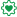# Qlik Sense App Development

Announcements
See why BI users voted Qlik #1 in 11 categories. GET REPORT
cancel
Showing results for
Did you mean:
HighlightedPartner

## Set Analysis Accumulation Not Working

Hello,

I have the following "As of" expression that is not showing the accumulation of the payment amount after the period when the payment first happened in 2012-Dec.  The set analysis syntax has a search parameter to include all payments on or before the  Max([Year Month]).  In this case the Max([Year Month]) is 2018-May.

Expression: Sum({<Year=,Month=,[Year Month]={"<= \$(=Max([Year Month]))"}>} PaymentAmount)

See attached screen shot of a table showing the payments.  The periods following 2012-Dec are zero, but should be 1,891.87.

Any information to resolve this issue would be greatly appreciated!

Thank you,

Labels (2)

• ### Set Analysis

1 Solution

Accepted Solutions
HighlightedMVP

Are you looking for this may be

```RangeSum(Above(
Sum({<Year=,Month=,[Year Month]={"<=\$(=Max([Year Month]))"}>} PaymentAmount)
, 0, RowNo()))```
5 Replies
HighlightedEmployee

The Max aggregation is for numeric fields, the Year month is a text string, so max will not work here

HighlightedMVP

It's better to deal with the string date fields in the script. However, if that is not in the scope, you could try parsing the string in set analysis itself like:

`Sum({<Year=,Month=,[Year Month]={"=Date#([Year Month], 'MMM-YYYY') <= \$(=Max(Date#([Year Month],'MMM-YYYY')))"}>} PaymentAmount) `
HighlightedPartner

Hi Lisa_P,

Thank you for the help!

The expression I am using is numeric.  However, to confirm if the "Year Month" was the issue, I changed the expression to the following.  The change did not correct the issue.  Please see attached screen shot of the table using the new expression.

Expression: Sum({<Year=,Month=,[_MonthSerial]={"<= \$(=Max([_MonthSerial]))"}>} PaymentAmount)

I would have thought the "< =" syntax in the expression would be able to show the balance forward to all future periods after the initial posting in Dec-2012.

HighlightedMVP

Are you looking for this may be

```RangeSum(Above(
Sum({<Year=,Month=,[Year Month]={"<=\$(=Max([Year Month]))"}>} PaymentAmount)
, 0, RowNo()))```
HighlightedPartner
Thanks Sunny!!